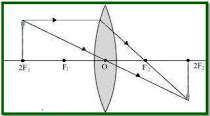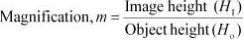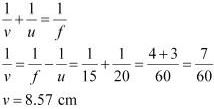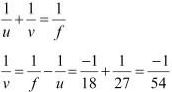# NCERT Solutions for Class 10 Science Chapter 10 Light- Reflection and Refraction

 Chapter Name NCERT Solutions for Class 10 Science Chapter 10 Light- Reflection and Refraction Topics Covered NCERT Intext QuestionsNCERT Exercise Solutions Related Study NCERT Solutions for Class 10 ScienceNCERT Revision Notes for Class 10 ScienceImportant Questions for Class 10 ScienceMCQ for Class 10 ScienceNCERT Exemplar Questions For Class 10 Science

### NCERT Intext Questions

Page 168

1. Define the principal focus of a concave mirror.

Solution

Light rays that are parallel to the principal axis of a concave mirror converge at a specific point on its principal axis after reflecting from the mirror. This point is known as the principal focus of the concave mirror.

2. The radius of curvature of a spherical mirror is 20 cm. What is its focal length?

Solution

Radius of curvature, R = 20 cm
Radius of curvature of a spherical mirror = 2 × Focal length (f)
R = 2f
f = R/2 = 20/2 = 10 cm
Hence, the focal length of the given spherical mirror is 10 cm.

3. Name the mirror that can give an erect and enlarged image of an object.

Solution

When an object is placed between the pole and the principal focus of a concave mirror, the image formed is virtual, erect, and enlarged.

4. Why do we prefer a convex mirror as a rear - view mirror in vehicles ?

Solution

Convex mirrors give a virtual, erect, and diminished image of the objects placed in from of them. They are preferred as a rear - view mirror in vehicles because they give a wider field of view, which allows the driver to see most of the traffic behind him.

Page 171

1. Find the focal length of a convex mirror whose radius of curvature is 32 cm.

Solution

Radius of curvature, R = 32 cm
Radius of curvature = 2 × Focal length (f)
R = 2f
f = R/2 = 32/2 = 16 cm
Hence, the focal length of the given convex mirror is 16 cm.

2. A concave mirror produces three times magnified (enlarged) real image of object placed at 10 cm in front of it. Where is the image located?

Solution

Magnification produced by a spherical mirror is given by the relation,
m = Height of the image/Height of the object = -(Image distance/Object distance)
⇒ m = h1/ho = -(v/u)
Let the height of the object, ho = h
Then, height of the image, h1 = -3h(Image formed is real)
-3h/h = -v/u
⇒ v/u = 3
Object distance, u = -10cm V = 3 × (-10) = -30 cm
Here, the negative sign indicates that an inverted image is formed at a distance of 30 cm in front of the given concave mirror.

Page 176

1. A ray of light travelling in air enters obliquely into water. Does the light ray bend towards the normal or away from the normal? Why?

Solution

The light ray bends towards the normal. When a ray of light travels from an optically rarer medium to an optically denser medium, it gets bent towards the normal. Since water is optically denser than air, a ray of light travelling from air into the water will bend towards the normal.

2. Light enters from air to glass having refractive index 1.50. What is the speed of light in the glass? The speed of light in vacuum is 3 × 108 ms-1 .

Solution

Refractive index of a medium nm is given by,
nm = (Speed of light in vacuum/Speed of light in the medium) = c/v
Speed of light in vacuum, c = 3 × 108 ms-1 .
Refractive index of glass, ng = 1.50
Speed of light in the glass, v = c/ng = 3 × 108 /1.50 = 2× 108 ms-1 .

3. Find out, from Table 10.3, the medium having highest optical density. Also find the medium with lowest optical density.
 Table 10.3 Absolute refractive index of some material media Material medium Refractive index Material medium Refractive index Air 1.0003 Canada Balsam 1.53 Ice 1.31 − − Water 1.33 Rock salt 1.54 Alcohol 1.36 − − Kerosene 1.44 Carbon 1.63 Fused 1.46 − − Quartz − Dense flint/glass − Turpentine oil 1.47 Ruby 1.71 Benzene 1.50 Sapphire 1.77 Crown glass 1.52 Diamond 2.42

Solution

Highest optical density = Diamond

Lowest optical density = Air
Optical density of a medium is directly related with the refractive index of that medium. A medium which has the highest refractive index will have the highest optical density and vice-versa.
It can be observed from table 10.3 that diamond and air respectively have the highest and lowest refractive index. Therefore, diamond has the highest optical density and air has the lowest optical density.

4. You are given kerosene, turpentine and water. In which of these does the light travel fastest? Use the information given in Table.

 Table 10.3 Absolute refractive index of some material media Material medium Refractive index Material medium Refractive index Air 1.0003 Canada Balsam 1.53 Ice 1.31 − − Water 1.33 Rock salt 1.54 Alcohol 1.36 − − Kerosene 1.44 Carbon 1.63 Fused 1.46 − − Quartz − Dense flint/glass − Turpentine oil 1.47 Ruby 1.71 Benzene 1.50 Sapphire 1.77 Crown glass 1.52 Diamond 2.42

Solution

Speed of light in a medium is given by the relation for refractive index (nm ). The relation is given as:

nm = (Speed of light in air / Speed of light in the medium) = c/v

⇒ v = c/nm
⇒ v ∝ 1/nm
It can be inferred from the relation that light will travel the slowest in the material which has the highest refractive index and travel the fastest in the material which has the lowest refractive index.
It can be observed from table 10.3 that the refractive indices of kerosene, turpentine, and water are 1.44, 1.47, and 1.33 respectively. Therefore, light travels the fastest in water.

5. The refractive index of diamond is 2.42. What is the meaning of this statement ?
Solution
Refractive index of a medium nm is related to the speed of light in that medium V by the relation :
nm = (Speed of light in air/Speed of light in the medium) = c/v
where, c is the speed of light in vacuum/air
The refractive index of diamond is 2.42. This suggests that the speed of light in diamond will reduce by a factor 2.42 compared to its speed in air.

Page 184

1. Define 1 dioptre of power of a lens.
Solution
Power of lens is defined as the reciprocal of its focal length. If P is the power of a lens of
focal length F in metres, then
P = 1/f(in metres)
The S.I. unit of power of a lens is Dioptre. It is denoted by D.
1 dioptre is defined as the power of a lens of focal length 1 metre.
∴ 1 D = 1 m-1

2. A convex lens forms a real and inverted image of a needle at a distance of 50 cm from it.
Where is the needle placed in front of the convex lens if the image is equal to the size of
the object? Also, find the power of the lens.
Solution
When an object is placed at the centre of curvature, 2F1, of a convex lens, its image is formed at the centre of curvature, 2F2, on the other side of the lens. The image formed is inverted and of the same size as the object, as shown in the given figure.It is given that the image of the needle is formed at a distance of 50 cm from the convex
lens. Hence, the needle is placed in front of the lens at a distance of 50 cm.
Object distance, u = −50 cm
Image distance, v = 50 cm
Focal length = f
According to the lens formula,

3. Find the power of a concave lens of focal length 2 m.
Solution
Focal length of concave lens, f = 2 m
Power of a lens, P = 1/f(in meters) = 1/(-50) = -0.5 D
Here, negative sign arises due to the divergent nature of concave lens.
Hence, the power of the given concave lens is -0.5 D.

### NCERT Exercises Solutions

1. Which one of the following materials cannot be used to make a lens ?
(a) Water
(b) Glass
(c) Plastic
(d) Clay
Solution
(d) A lens allows light to pass through it. Since clay does not show such property, it cannot be used to make a lens.

2. The image formed by a concave mirror is observed to be virtual, erect and larger than the object. Where should be the position of the object ?
(a) Between the principal focus and the centre of curvature
(b) At the centre of curvature
(c) Beyond the centre of curvature
(d) Between the pole of the mirror and its principle focus.
Solution
(d) When an object is placed between the pole and principal focus of a concave mirror, the image formed is virtual, erect, and larger than the object .

3. Where should an object be placed in front of a convex lens to get a real image of the size of the object ?
(a) At the principal focus of the lens
(b) At twice the focal length at infinity
(c) Between the optical centre of the lens and its principal focus.
Solution
(b) When an object is placed at the centre of curvature in front of a convex lens, its image is formed at the centre of curvature on the other side of the lens. The image formed is real, inverted, and of the same size as the object.

4. A spherical mirror and a thin spherical lens have each a focal length of −15 cm. The mirror
and the lens are likely to be
(a) both concave
(b) both convex
(c) the mirror is concave and the lens is convex
(d) the mirror is convex, but the lens is concave
Solution
By convention, the focal length of a concave mirror and a concave lens are taken as negative. Hence, both the spherical mirror and the thin spherical lens are concave in nature.

5. No matter how far you stand from a mirror, your image appears erect. The mirror is likely
to be
(a) plane
(b) concave
(c) convex
(d) either plane or convex
Solution
(d) A convex mirror always gives a virtual and erect image of smaller size of the object
placed in front of it. Similarly, a plane mirror will always give a virtual and erect image of
same size as that of the object placed in front of it. Therefore, the given mirror could be
either plane or convex.

6. Which of the following lenses would you prefer to use while reading small letters found in
a dictionary?
(a) A convex lens of focal length 50 cm
(b) A concave lens of focal length 50 cm
(c) A convex lens of focal length 5 cm
(d) A concave lens of focal length 5 cm
Solution
(c) A convex lens gives a magnified image of an object when it is placed between the radius of curvature and focal length. Also, magnification is more for convex lenses having shorter focal length. Therefore, for reading small letters, a convex lens of focal length 5 cm should be used.

7. We wish to obtain an erect image of an object, using a concave mirror of focal length 15 cm. What should be the range of distance of the object from the mirror? What is the nature of the image? Is the image larger or smaller than the object? Draw a ray diagram to show the image formation in this case.
Solution
Range of object distance = 0 cm to15 cm
A concave mirror gives an erect image when an object is placed between its pole (P) and the principal focus (F).
Hence, to obtain an erect image of an object from a concave mirror of focal length 15 cm, the object must be placed anywhere between the pole and the focus. The image formed will be virtual, erect, and magnified in nature, as shown in the given figure.

8. Name the type of mirror used in the following situations.
(b) Side/rear-view mirror of a vehicle
(c) Solar furnace
Solution
(a) Concave
(b) Convex
(c) Concave
Explanation
(a) Concave mirror is used in the headlights of a car. This is because concave mirrors can produce powerful parallel beam of light when the light source is placed at their principal focus.
(b) Convex mirror is used in side/rear view mirror of a vehicle. Convex mirrors give a virtual, erect, and diminished image of the objects placed in front of it. Because of this, they have a wide field of view. It enables the driver to see most of the traffic behind him/her.
(c) Concave mirrors are convergent mirrors. That is why they are used to construct solar furnaces. Concave mirrors converge the light incident on them at a single point known as principal focus. Hence, they can be used to produce a large amount of heat at that point.

9. One-half of a convex lens is covered with a black paper. Will this lens produce a complete image of the object? Verify your answer experimentally. Explain your observations.
Solution
The convex lens will form complete image of an object, even if its one half is covered with black paper. It can be understood by the following two cases.
Case I
When the upper half of the lens is covered In this case, a ray of light coming from the object will be refracted by the lower half of the lens. These rays meet at the other side of the lens to form the image of the given object, as shown in the following figure.Case II
When the lower half of the lens is covered In this case, a ray of light coming from the object is refracted by the upper half of the lens. These rays meet at the other side of the lens to form the image of the given object, as shown in the following figure.

10. An object 5 cm in length is held 25 cm away from a converging lens of focal length 10 cm.
Draw the ray diagram and find the position, size and the nature of the image formed.
Solution
Object distance, u = −25 cm
Object height, ho = 5 cm
Focal length, f = +10 cm
According to the lens formula,
1/v - 1/u = 1/f
⇒ 1/v = 1/f + 1/u
⇒ 1/10 - 1/25 = 15/250
⇒ v = 250/15 = 16.66 cm
The positive value of v shows that the image is formed at the other side of the lens.
Magnification, m = -(Image distance/Object distance) = -v/u = -16.66/25 = -0.66
The negative sign shows that the image is real and formed behind the lens.
Magnification, m = Image height/Object height = H1/Ho = H1/5
H1 = m × Ho = -0.66 × 5 = -3.3 cm
The negative value of image height indicates that the image formed is inverted.
The position, size, and nature of image are shown in the following ray diagram.

11. A concave lens of focal length 15 cm forms an image 10 cm from the lens. How far is the object placed from the lens? Draw the ray diagram.
Solution
Focal length of concave lens (OF1), f = −15 cm
Image distance, v = −10 cm

13. The magnification produced by a plane mirror is +1. What does this mean?
Solution
Magnification produced by a mirror is given by the relationThe magnification produced by a plane mirror is +1. It shows that the image formed by the plane mirror is of the same size as that of the object. The positive sign shows that the image formed is virtual and erect.

14. An object 5.0 cm in length is placed at a distance of 20 cm in front of a convex mirror of radius of curvature 30 cm. Find the position of the image, its nature and size.
Solution
Object distance, u = −20 cm
Object height, h = 5 cm
Radius of curvature, R = 30 cm
Radius of curvature = 2 × Focal length
R = 2f f = 15 cm
According to the mirror formula,The positive value of v indicates that the image is formed behind the mirror.
Magnification, m = - (Image distance/Object distance) = -8.57/-20 = 0.428
The positive value of magnification indicates that the image formed is virutal.
Magnification, m = Height of the image/Height of the object = h'/h
h' = m × h = 0.428 × 5= 2.14 cm
The positive value of image height indicates that the image formed is erect.
Therefore, the image formed is virtual, erect, and smaller in size.

15. An object of size 7.0 cm is placed at 27 cm in front of a concave mirror of focal length 18 cm. At what distance from the mirror should a screen be placed, so that a sharp focused image can be obtained? Find the size and the nature of the image.
Solution
Object distance, u = −27 cm
Object height, h = 7 cm
Focal length, f = −18 cm
According to the mirror formula,v = -54 cm
The screen should be placed at a distance of 54 cm in front of the given mirror.
Magnification, m = -(Image distance/Object distance) = -54/27 = -2
The negative value of magnification indicates that the image formed is real.
Magnification, m = (Height of the image/Height of the object) = h'/h
h' = 7 × (-2) = -14 cm
The negative value of image height indicates that the image formed is inverted.

16. Find the focal length of a lens of power −2.0 D. What type of lens is this?
Solution
Power of a lens, P = 1/f(in metres)
P = -2 D
f = -1/2 = -0.5 m
A concave lens has a negative focal length. Hence, it is a concave lens.

17. A doctor has prescribed a corrective lens of power +1.5 D. Find the focal length of the lens. Is the prescribed lens diverging or converging?
Solution
Power of lens, P = 1/f(in metres)
Power, P = 1.5 D
f = 1/1.5 = 10/15 = 0.66 m
A convex lens has a positive focal length. Hence, it is a convex lens or a converging  lens.